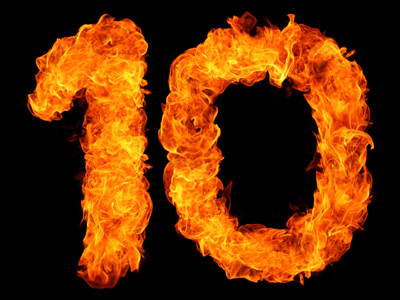Multiplication and division are easy if they involve multiples of ten.

# Multiplication and Division 2 (Difficult)

So, you’ve made it to the second of our difficult level Eleven Plus maths quizzes on multiplication and division. If you’ve played all the previous quizzes in this section you should be a dab hand at multiplying and dividing numbers by now – we’ll soon find out!

In the last quiz we concentrated on multiplication and division involving multiples of ten such as, 100, 1,000, 10,000 and 100,000. Large numbers, but all very easy to work with – all you have to do is move the digits the correct number of places in the correct direction (right or left). But I think you are ready for more of a challenge so, in this quiz, we’ll be dealing with large numbers which are not multiples of ten.

I’m sure you mastered long division and long multiplication long ago, so you could do these without any problem with the help of pencil and paper. Instead, try working these answers out in your head, using some of the techniques we’ve learned so far.

So, if you are ready, let’s begin. Good luck!

1.
What is the correct answer to the given calculation?
84,450 ÷ 2,000 = ?
45.222
42.225
42.252
42.522
To divide a number by 2,000 just divide the number by 2, then by 1,000 – simple!
2.
What is the correct answer to the given calculation?
14.369 × 210 = ?
3,019.74
3,047.19
3,014.79
3,017.49
To multiply a number by 210, first multiply by 2 and then by 100, next multiply the original number by 10 and add your two answers together:
14.369 × 210 = ((14.369 x 2) x 100) + (14.369 x 10) = (28.738 x 100) + 143.69 = 2,873.8 + 143.69 = 3,017.49
3.
What is the correct answer to the given calculation?
27.3 × 98 = ?
267.54
26,754
2,675.4
26.754
To multiply a number by 98, first multiply the number by 100, then subtract double the original number:
27.3 × 98 = (27.3 x 100) - (27.3 x 2) = 2,730 - 54.6 = 2,675.4
4.
What is the correct answer to the given calculation?
1.532 × 104 = ?
159.328
159.238
159.832
159.382
To multiply a number by 104, first multiply the number by 100, then add quadruple the original number to it:
1.532 × 104 = (1.532 x 100) + (1.532 x 4) = 153.2 + 6.128 = 159.328
5.
What is the correct answer to the given calculation?
14,550 ÷ 300 = ?
45.8
48.5
54.8
58.4
To divide a number by 300: first divide by 3, then divide by 100:
14,550 ÷ 300 = (14,550 ÷ 3) ÷ 100 = 4,850 ÷ 100 = 48.5
6.
What is the correct answer to the given calculation?
342 ÷ 15 = ?
28.2
26.2
22.8
24.8
To divide a number by 15, first divide by 10, then multiply by 2, and finally divide by 3:
342 ÷ 15 = ((342 ÷ 10) x 2) ÷ 3 = (34.2 x 2) ÷ 3 = 68.4 ÷ 3 = 22.8
7.
What is the correct answer to the given calculation?
128.2 × 1,005 = ?
128,481
128,148
128,814
128,841
To multiply a number by 1,005: first multiply by 1,000, then divide the original number by 2 and move the digits to the left, finally add these two numbers together:
128.2 × 1,005 = (128.2 x 1,000) + ((128.2 ÷ 2) x 10) = 128,200 + (64.1 x 10) = 128,200 + 641 = 128,841
8.
What is the correct answer to the given calculation?
350,000 ÷ 9,999 = ?
35.305
35.035
35.35
35.0035
To divide a number by 9,999, here’s a neat trick - divide by both 10,000 and 100,000,000 and add the two answers together:
350,000 ÷ 9,999 = (350,000 ÷ 10,000) + (350,000 ÷ 100,000,000) = 35 + 0.0035 = 35.0035
9.
What is the correct answer to the given calculation?
16,320 ÷ 1,020 = ?
16
17
18
19
This is a toughie but you can get through it with trial and error
10.
What is the correct answer to the given calculation?
11,002 × 150 = ?
1,560,300
1,650,300
1,350,600
1,630,500
To multiply a number by 150, first divide by 2, then move the digits 2 places to the left, then multiply the original number by 100, finally add these two answers together:
11,002 × 150 = ((11,002 ÷ 2) x 100) + 11,002 x 100 = (5,501 x 100) + 1,100,200 = 550,100 + 1,100,200 = 1,650,300
Author:  Frank Evans